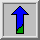Common Names: Pixel Add, Sum, Offset

## Brief Description

In its most straightforward implementation, this operator takes as input two identically sized images and produces as output a third image of the same size as the first two, in which each pixel value is the sum of the values of the corresponding pixel from each of the two input images. More sophisticated versions allow more than two images to be combined with a single operation.

A common variant of the operator simply allows a specified constant to be added to every pixel.

## How It Works

The addition of two images is performed straightforwardly in a single pass. The output pixel values are given by: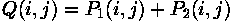Or if it is simply desired to add a constant value C to a single image then: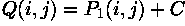If the pixel values in the input images are actually vectors rather than scalar values (e.g. for color images) then the individual components (e.g. red, blue and green components) are simply added separately to produce the output value.

If the image format being used only supports, say 8-bit integer pixel values, then it is very easy for the result of the addition to be greater than the maximum allowed pixel value. The effect of this depends upon the particular implementation. The overflowing pixel values might just be set to the maximum allowed value, an effect known as saturation. Alternatively the pixel values might wrap around from zero again. If the image format supports pixel values with a much larger range, e.g. 32-bit integers or floating point numbers, then this problem does not occur so much.## Guidelines for Use

Image addition crops up most commonly as a sub-step in some more complicated process rather than as a useful operator in its own right. As an example we show how addition can be used to overlay the output from an edge detector on top of the original image after suitable masking has been carried out.

The imageshows a simple flat dark object against a light background. Applying the Canny edge detector to this image, we obtain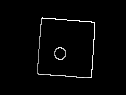Suppose that our task is to overlay this edge data on top of the original image. The image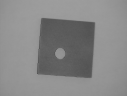is the result of straightforwardly adding the two images. Since the sum of the edge pixels and the underlying values in the original is greater than the maximum possible pixel value, these pixels are (in this implementation) wrapped around. Therefore these pixels have a rather low pixel value and it is hard to distinguish them from the surrounding pixels. In order to avoid the pixel overflow we need to replace pixels in the original image with the corresponding edge data pixels, at every place where the edge data pixels are non-zero. The way to do this is to mask off a region of the original image before we do any addition.

The mask is made by thresholding the edge data at a pixel value of 128 in order to produce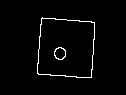This mask is then inverted and subsequently ANDed with the original image to produceFinally, the masked image is added to the unthresholded edge data to produce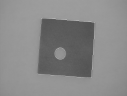This image now clearly shows that the Canny edge detector has done an extremely good job of localizing the edges of the original object accurately. It also shows how the response of the edge detector drops off at the fuzzier left hand edge of the object.

Other uses of addition include adding a constant offset to all pixels in an image so as to brighten that image. For example, adding a constant value of 50 to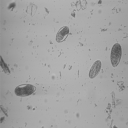yields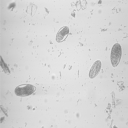It is important to realize that if the input images are already quite bright, then straight addition may produce a pixel value overflow. Image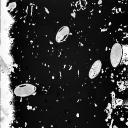shows the results of adding 100 to the above image. Most of the background pixels are greater than the possible maximum (255) and therefore are (with this implementation of addition) wrapped around from zero. If we implement the operator in such a way that pixel values exceeding the maximum value are set to 255 (i.e. using a hard limit) we obtain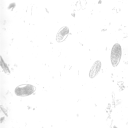This image looks more natural than the wrapped around one. However, due to the saturation, we lose a certain amount of information, since all the values exceeding the maximum value are set to the same graylevel.

In this case, the pixel values should be scaled down before addition. The image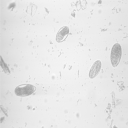is the result of scaling the original with 0.8 and adding a constant value of 100. Although the image is brighter than the original, it has lost contrast due to the scaling. In most cases, scaling the image with a factor larger than 1 without using addition at all provides a better way to brighten an image, as it increases the image contrast. For comparison,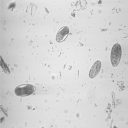is the original image multiplied with 1.3.

Blending provides a slightly more sophisticated way of merging two images which ensures that saturation cannot happen.

When adding color images it is important to consider how the color information has been encoded. The section on 8-bit color images describes the issues to be aware of when adding such images.

## Interactive Experimentation

You can interactively experiment with this operator by clicking here.

## Exercises

1. Add the above Canny edge image to its original, using different implementation's of pixel addition which handle the pixel overflow in different ways. Which one yields the best results for this implementation?
2. Use skeletonization to produce a skeleton ofAdd the skeleton to the original. Which problems do you face and how might they be solved?

3. Add a constant value of 255 toUse two different implementations, one wrapping around from zero all pixel values exceeding the maximum value and one using a hard limit of 255. Comment on the results.

## References

A. Marion An Introduction to Image Processing, Chapman and Hall, 1991, pp 242 - 244.

D. Vernon Machine Vision, Prentice-Hall, 1991, pp 51 - 52.

## Local Information